## SMARTTRIM

 Automatically trims an image around the region of highest detail in the image.

### EXAMPLES

 Example 1 --- Comparison Of Metrics For Fuzzvals 1,2,5,10,15 And Given Graymode and Restrict Options Original (original)Arguments: -g grayscale -r no -g grayscale -r no -g grayscale -r no -g grayscale -r no (-f fuzzvals of 1, 2, 5, 10, 15 left to right) sobelstd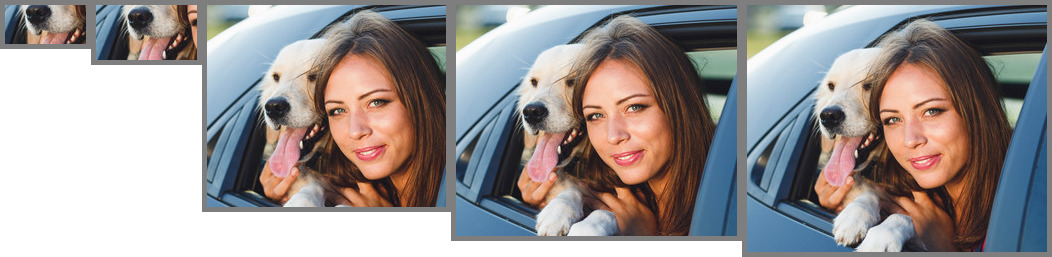cannycornersArguments: -g maximum -r no -g maximum -r no -g maximum -r no -g maximum -r no (-f fuzzvals of 1, 2, 5, 10, 15 left to right) sobelstdcanny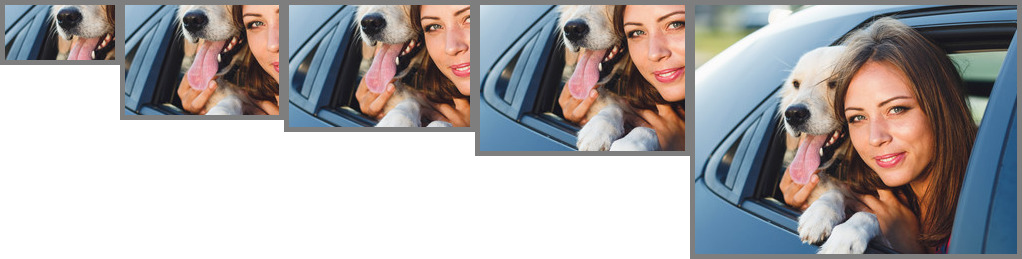cornersArguments: -g grayscale -r yes -g grayscale -r yes -g grayscale -r yes -g grayscale -r yes (-f fuzzvals of 1, 2, 5, 10, 15 left to right) sobelstdcannycorners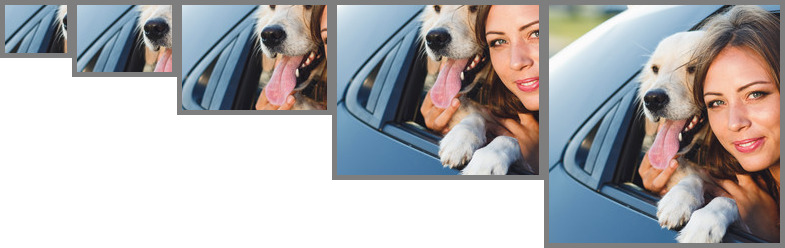Arguments: -g maximum -r yes -g maximum -r yes -g maximum -r yes -g maximum -r yes (-f fuzzvals of 1, 2, 5, 10, 15 left to right) sobel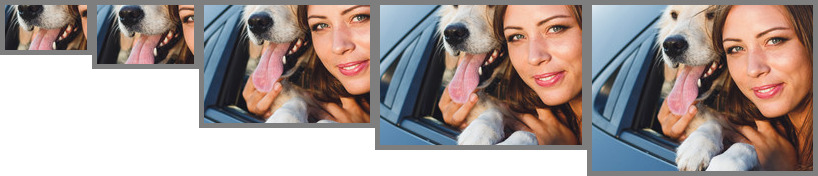std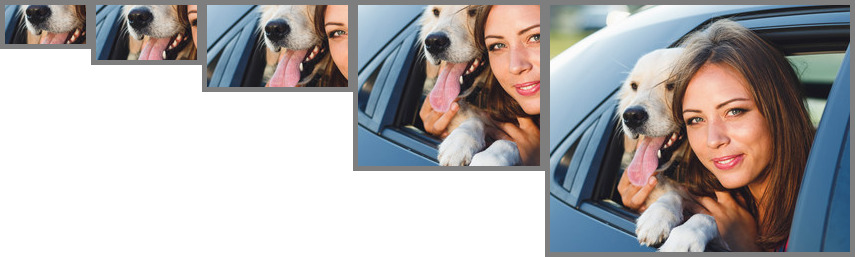cannycornersExample 2 --- Comparison Of Metrics For Fuzzvals 1,2,5,10,15 And Given Graymode and Restrict Options Original (original)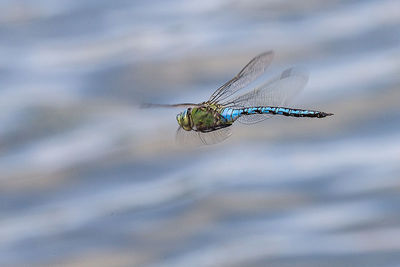Arguments: -g grayscale -r no -g grayscale -r no -g grayscale -r no -g grayscale -r no (-f fuzzvals of 1, 2, 5, 10, 15 left to right) sobel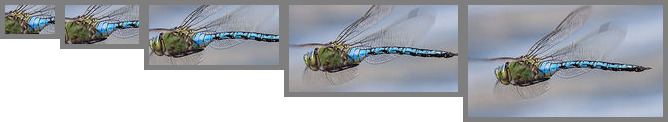std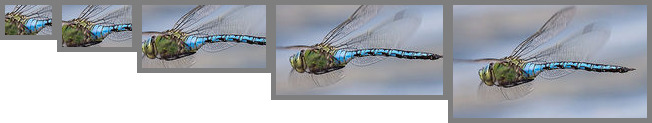cannycornersArguments: -g maximum -r no -g maximum -r no -g maximum -r no -g maximum -r no (-f fuzzvals of 1, 2, 5, 10, 15 left to right) sobel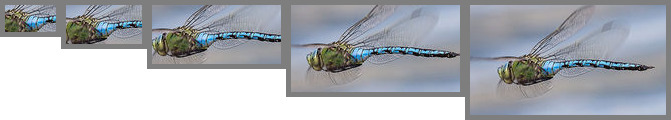stdcannycornersArguments: -g grayscale -r yes -g grayscale -r yes -g grayscale -r yes -g grayscale -r yes (-f fuzzvals of 1, 2, 5, 10, 15 left to right) sobelstdcannycorners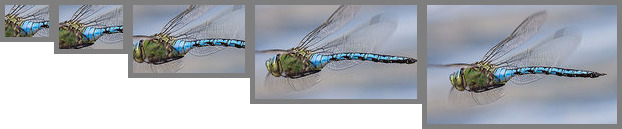Arguments: -g maximum -r yes -g maximum -r yes -g maximum -r yes -g maximum -r yes (-f fuzzvals of 1, 2, 5, 10, 15 left to right) sobelstdcannycornersExample 3 --- Comparison Of Metrics For Fuzzvals 1,2,5,10,15 And Given Graymode and Restrict Options Original (original)Arguments: -g grayscale -r no -g grayscale -r no -g grayscale -r no -g grayscale -r no (-f fuzzvals of 1, 2, 5, 10, 15 left to right) sobelstdcanny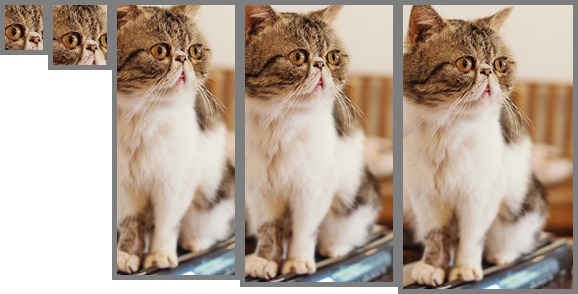cornersArguments: -g maximum -r no -g maximum -r no -g maximum -r no -g maximum -r no (-f fuzzvals of 1, 2, 5, 10, 15 left to right) sobelstdcannycornersArguments: -g grayscale -r yes -g grayscale -r yes -g grayscale -r yes -g grayscale -r yes (-f fuzzvals of 1, 2, 5, 10, 15 left to right) sobel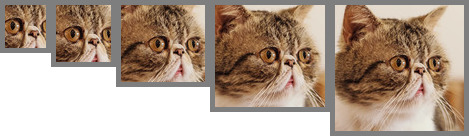stdcanny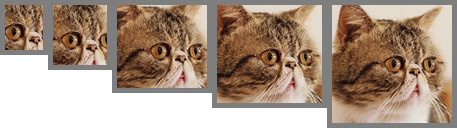corners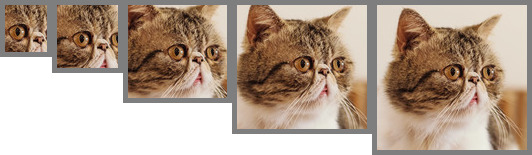Arguments: -g maximum -r yes -g maximum -r yes -g maximum -r yes -g maximum -r yes (-f fuzzvals of 1, 2, 5, 10, 15 left to right) sobel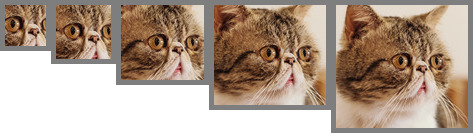std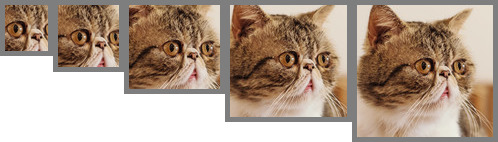cannycornersExample 4 --- Comparison Of Metrics For Fuzzvals 1,2,5,10,15 And Given Graymode and Restrict Options Original (original)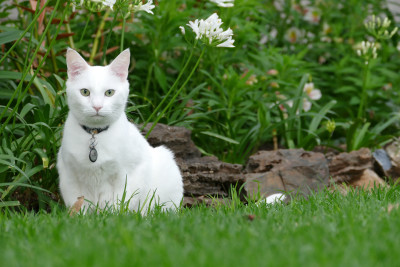Arguments: -g grayscale -r no -g grayscale -r no -g grayscale -r no -g grayscale -r no (-f fuzzvals of 1, 2, 5, 10, 15 left to right) sobelstdcannycornersArguments: -g maximum -r no -g maximum -r no -g maximum -r no -g maximum -r no (-f fuzzvals of 1, 2, 5, 10, 15 left to right) sobel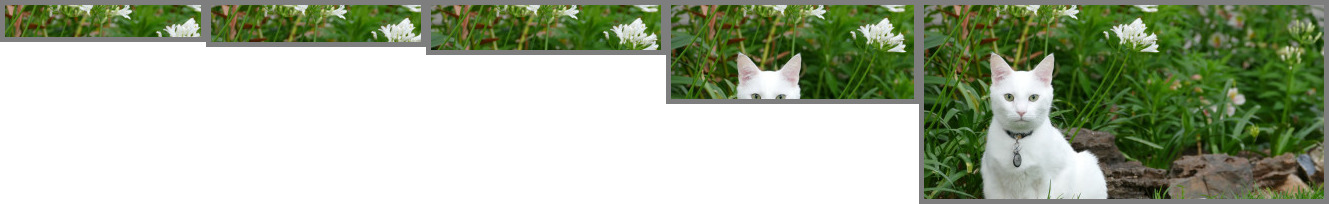stdcanny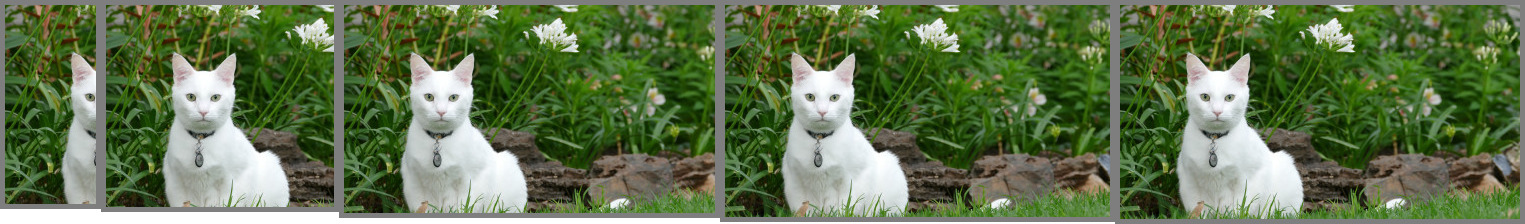corners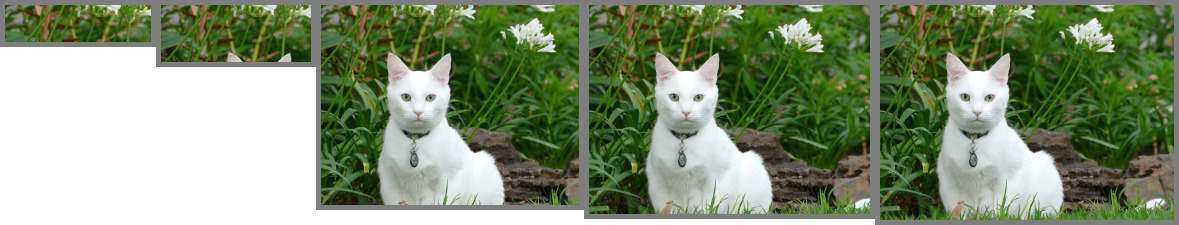Arguments: -g grayscale -r yes -g grayscale -r yes -g grayscale -r yes -g grayscale -r yes (-f fuzzvals of 1, 2, 5, 10, 15 left to right) sobel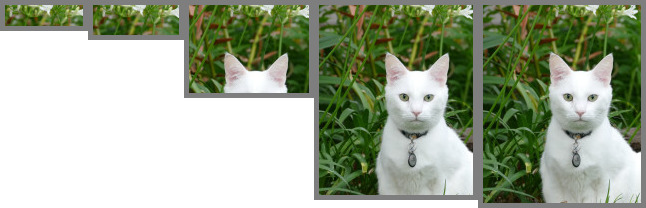std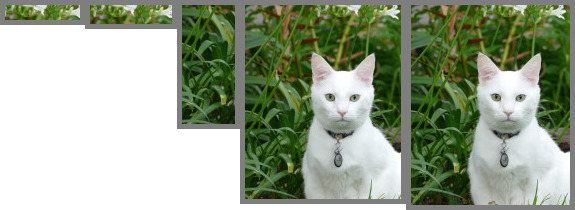cannycornersArguments: -g maximum -r yes -g maximum -r yes -g maximum -r yes -g maximum -r yes (-f fuzzvals of 1, 2, 5, 10, 15 left to right) sobelstdcanny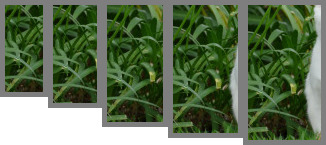corners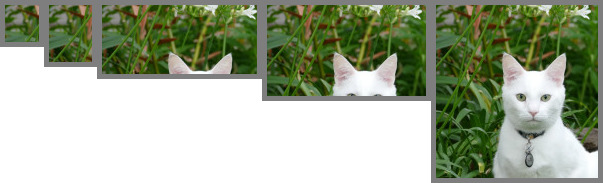Example 5 --- Comparison Of Blur Values Original (original)Arguments: -g grayscale -r no -f 2 (-b blur 20, 30, 40 left to right)Example 6 --- Comparison Of Different Crop Sizes Arguments: -m sobel -g grayscale -r no -f 5 -b 20 (-s sizes automatic, 50x50, 100x50, 200x100, 200x150, 200x200, 250x150 left to right)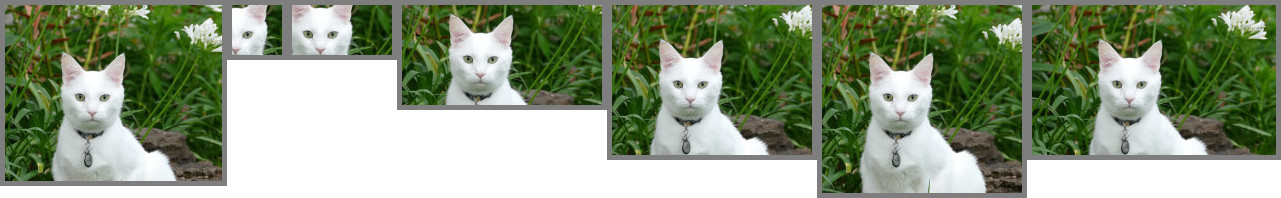Example 7 --- Trim Of Text Scan Original (original)Arguments: -m corners -g grayscale -r no -f 10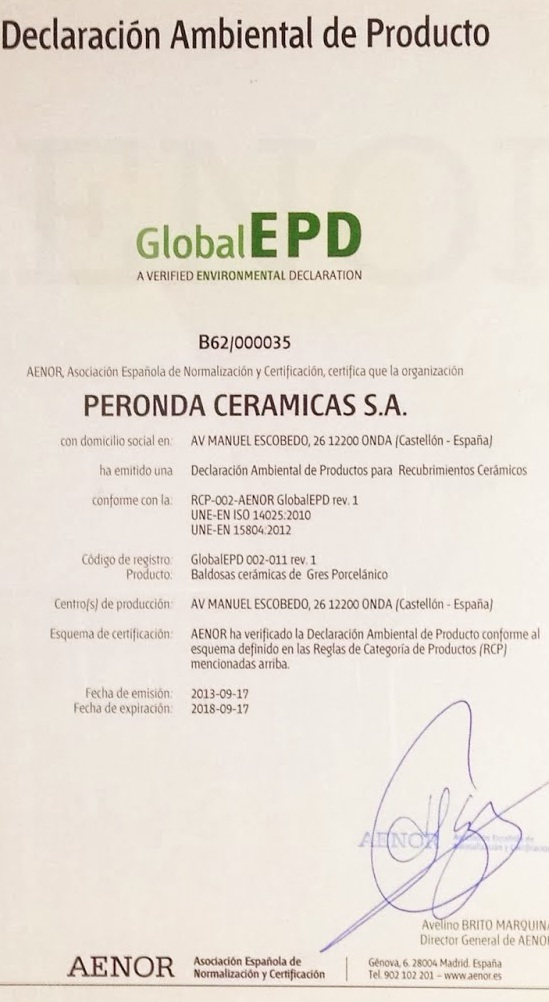Example 8 --- Trim Of Text Scan Original (original)Arguments: -m corners -g grayscale -r no -f 8Example 9 --- Trim Of Text Scan Original (original)Arguments: -m corners -g grayscale -r no -f 30Example 10 --- Trim Of Text Scan Original (original)Arguments: -m corners -g grayscale -r no -f 55What the script does is as follows for the case of -r no: Reads the input Applies the desired metric, fuzzval, graymode and blur Equalizes the image and thresholds it according to 100-fuzzval Extracts the the trim coordinates Crops the input according to the trim coordinates Writes the output This is equivalent to the following IM commands if [ "\$metric" = "std" ]; then dproc="-statistic standard_deviation 3x3 -colorspace gray" elif [ "\$metric" = "sobel" ]; then dproc="-define convolve:scale=50%! -bias 50% -write mpr:img +delete \ mpr:img -morphology Convolve Sobel:0 +write mpr:dx +delete \ mpr:img -morphology Convolve Sobel:90 +write mpr:dy +delete \ mpr:dx mpr:dy -solarize 50% -level 50,0% -evaluate Pow 2 \ -compose plus -composite -evaluate Pow 0.5" elif [ "\$metric" = "canny" ]; then dproc="-canny 0x1+\${lowerthresh}%+\${upperthresh}%" elif [ "\$metric" = "corners" ]; then dproc="-write mpr:img +delete \ mpr:img -morphology dilate plus:1 -morphology erode diamond:1 -write mpr:plus +delete \ mpr:img -morphology dilate cross:1 -morphology erode square:1 -write mpr:cross +delete \ mpr:plus mpr:cross -compose difference -composite" fi if [ "\$graymode" = "grayscale" ]; then gproc="-colorspace gray" elif [ "\$graymode" = "maximum" ]; then gproc="-separate -evaluate-sequence max" fi if [ "\$blur" != "0" ]; then bproc="-blur 0x\$blur" else bproc="" fi thresh=`convert xc: -format "%[fx:100-\$fuzzval]" info:` cropvals=`convert "\$infile" -alpha off \ \$dproc \$gproc \$bproc -equalize -threshold \$thresh% \ -format "%@" info:` convert "\$infile" -crop \$cropvals +repage "\$outfile"